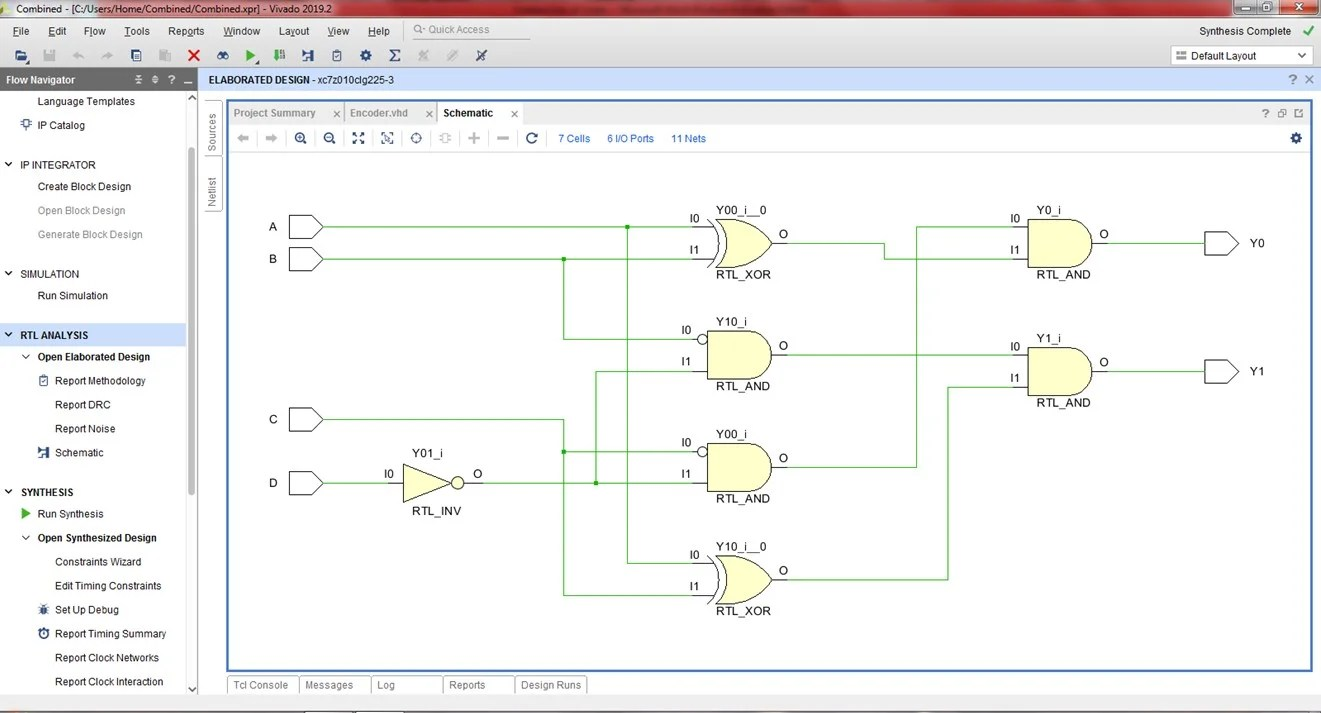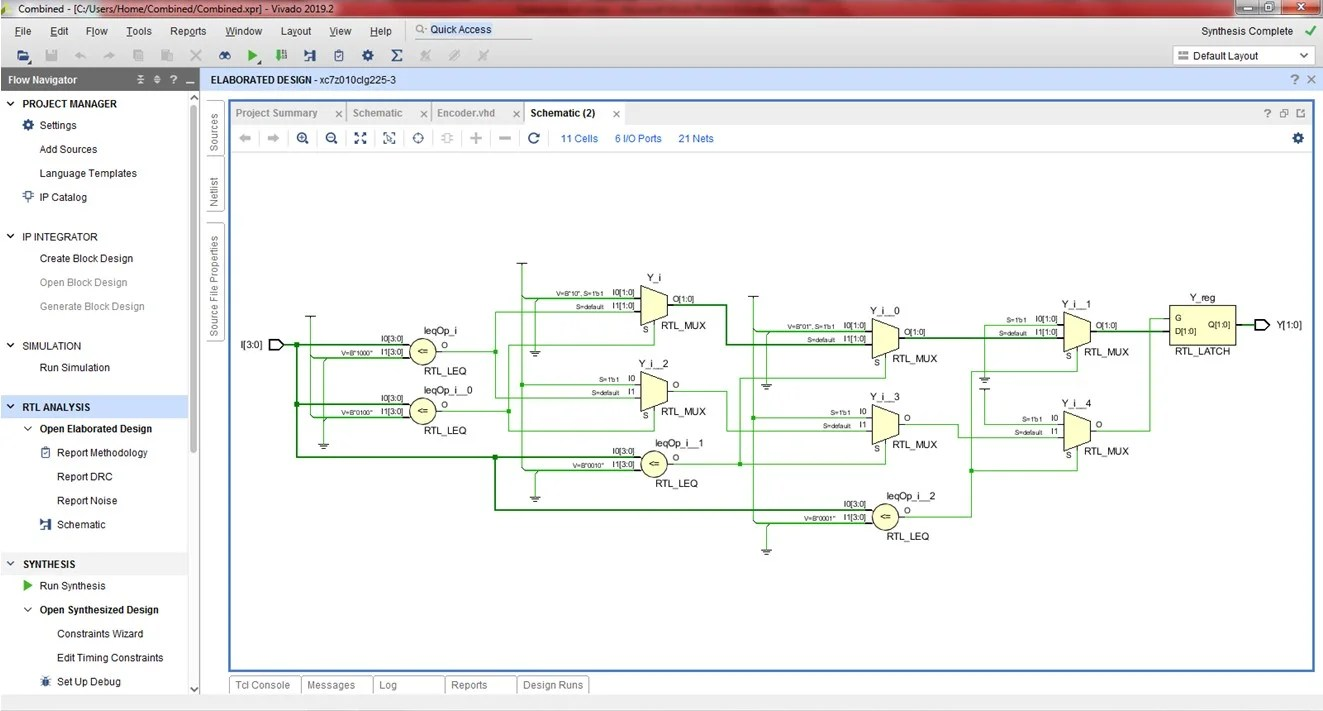View Course Path

# VHDL code for an encoder using dataflow method – full code and explanation

In this post, we will take a look at implementing the VHDL code for an encoder using dataflow modeling architecture. We will use two different approaches to help you understand the different possibilities of coding using the same modeling style. We will write a separate testbench for each method. We will also observe the resulting difference in the RTL schematics. Let’s start off by revising the 4×2 encoder circuit first.

Contents

## 4×2 Encoder

An encoder is a combinational logic circuit that takes in multiple inputs, encodes them, and outputs an encoded version with fewer bits. In a simple encoder, only one of the input lines is active at any moment. You can revisit the encoder circuit and its working here. In this post, we will write the VHDL code for a 4:2 encoder using its logic equations and its truth table.

### Logic circuit of a 4:2 encoder

A 4:2 encoder has four input ports and two output ports. That’s all the information we need to declare our entity-architecture pair for the VHDL program.The logic equations for the above circuit are as follows.

Y0 = A’BC’D’ + AB’C’D’ = C’D'($A \oplus B$)

Y1 = A’B’CD’ + AB’C’D’ = B’D'($A \oplus C$)

### Truth table of a 4:2 encoder

 A B C D Y0 Y1 0 0 0 1 0 0 0 0 1 0 0 1 0 1 0 0 1 0 1 0 0 0 1 1

## Using logic equation

### Explanation of the VHDL code for an encoder using dataflow method. How does the code work?

Let’s start with writing the VHDL code for the encoder using its logic equations. First off, we will write the code for the entity-architecture pair. We will declare the input and output ports as simple, discrete `STD_LOGIC` entities. The name of our entity, chosen by us, is `ENCODER_SOURCE`. We are using the dataflow architecture.

```entity ENCODER_SOURCE is
Port ( A,B,C,D : in  STD_LOGIC;
Y0,Y1 : out  STD_LOGIC);
end ENCODER_SOURCE;

architecture dataflow of ENCODER_SOURCE is

begin
```

And then we use the logic equations we saw above and replace the ‘=’ sign with the assignment operator of VHDL, ‘<=’.

```Y0 <= ((not C)and(not D))and(A xor B);
Y1 <= ((not B)and(not D))and(A xor C);

end dataflow;```

### Full VHDL code for an encoder using dataflow method – via logic equations

```library IEEE;
use IEEE.STD_LOGIC_1164.ALL;
use IEEE.STD_LOGIC_ARITH.ALL;
use IEEE.STD_LOGIC_UNSIGNED.ALL;

entity ENCODER_SOURCE is
Port ( A,B,C,D : in  STD_LOGIC;
Y0,Y1 : out  STD_LOGIC);
end ENCODER_SOURCE;

architecture dataflow of ENCODER_SOURCE is

begin

Y0 <= ((not C)and(not D))and(A xor B);
Y1 <= ((not B)and(not D))and(A xor C);

end dataflow;```

### Testbench

You can learn how to write a testbench using VHDL here.

```library IEEE;
use IEEE.STD_LOGIC_1164.ALL;
use IEEE.STD_LOGIC_ARITH.ALL;
use IEEE.STD_LOGIC_UNSIGNED.ALL;

entity encoder_dataflow_tb is
end entity;

architecture tb of encoder_dataflow_tb is
component ENCODER_SOURCE is
Port ( A,B,C,D : in STD_LOGIC;
Y0,Y1 : out STD_LOGIC);
end component;
signal A, B, C, D, Y0, Y1 : STD_LOGIC;

begin

uut: ENCODER_SOURCE port map(
A => A, B => B,
C => C, D => D,
Y0 => Y0, Y1 => Y1);

stim: process
begin

A <= '0';
B <= '0';
C <= '0';
D <= '1';
wait for 20 ns;

A <= '0';
B <= '0';
C <= '1';
D <= '0';
wait for 20 ns;

A <= '0';
B <= '1';
C <= '0';
D <= '0';
wait for 20 ns;

A <= '1';
B <= '0';
C <= '0';
D <= '0';
wait for 20 ns;

wait;

end process;
end tb;```

### RTL Schematic### Simulation Waveforms## Using Truth Table

### Explanation of the VHDL code for an encoder using dataflow method via truth table. How does the code work?

For the VHDL code of the encoder using its truth tables and the dataflow architecture, we will use the when-else statements that we studied in the dataflow architecture post.

The syntax is simple; we assign the output to a particular value and mention the condition followed by else statements. We will see the syntax for that shortly.

First, let’s declare the entity-architecture pair.

In this case, we will be defining the ports as vector quantities. As we have seen in many of our previous posts in this VHDL tutorial course, when dealing with truth tables, we have multiple inputs and generally multiple outputs too. In these cases, defining the ports as vector quantities gives us the room to write cleaner and more systematic code.

```entity ENCODER_SOURCE is
Port ( I : in  STD_LOGIC_VECTOR (3 downto 0);
Y : out  STD_LOGIC_VECTOR (1 downto 0));
end ENCODER_SOURCE;

architecture dataflow of ENCODER_SOURCE is

begin
```

And then we begin (with the begin command) to define the relationship between the entities using when-else statements. The syntax is shown below.

```Y <=       "00" when I <= "0001"
else "01" when I <= "0010"
else "10" when I <= "0100"
else "11" when I <= "1000";

end dataflow;```

### Full VHDL code for an encoder using dataflow method – via truth tables

```library IEEE;
use IEEE.STD_LOGIC_1164.ALL;
use IEEE.STD_LOGIC_ARITH.ALL;
use IEEE.STD_LOGIC_UNSIGNED.ALL;

entity ENCODER_SOURCE is
Port ( I : in  STD_LOGIC_VECTOR (3 downto 0);
Y : out  STD_LOGIC_VECTOR (1 downto 0));
end ENCODER_SOURCE;

architecture dataflow of ENCODER_SOURCE is

begin

Y <=       "00" when I <= "0001"
else "01" when I <= "0010"
else "10" when I <= "0100"
else "11" when I <= "1000";

end dataflow;```

### Testbench

```library IEEE;
use IEEE.STD_LOGIC_1164.ALL;
use IEEE.STD_LOGIC_ARITH.ALL;
use IEEE.STD_LOGIC_UNSIGNED.ALL;

entity encoder_tb is
end entity;

architecture tb of encoder_tb is
component ENCODER_SOURCE is
Port ( I : in STD_LOGIC_VECTOR (3 downto 0);
Y : out STD_LOGIC_VECTOR (1 downto 0));
end component;

signal I : STD_LOGIC_VECTOR (3 downto 0);
signal Y : STD_LOGIC_VECTOR (1 downto 0);

begin

uut: ENCODER_SOURCE port map(
I => I,
Y => Y);

stim: process
begin

I <= "0001";
wait for 20 ns;

I <= "0010";
wait for 20 ns;

I <= "0100";
wait for 20 ns;

I <= "1000";
wait for 20 ns;

wait;

end process;
end tb;```

### RTL Schematic### Simulation WaveformsHere’s an assignment for you. Now that you have written the VHDL code for an encoder try writing one for a priority encoder. It isn’t tough. Check out the link to brush up on the working of the priority encoder and get to work!

Edit: Post updated with the testbench, RTL Schematic, and Simulation Waveform by Deepak Joshi.

This site uses Akismet to reduce spam. Learn how your comment data is processed.